Year 8 Interactive Maths - Second Edition

## Area of a Circle

### Activity 12.1

Equipment:  You will need a compass, pair of scissors, ruler and protractor for this activity.
Purpose:
To discover a formula for the area of a circle.

Step 1:  Using the compass, draw a circle of radius 7 cm.  Then mark the circle's centre and draw its radius.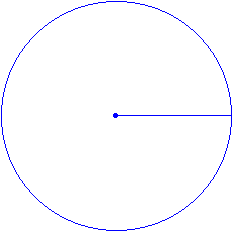Step 2:  Place the centre of the protractor at the centre of the circle and the zero line along the radius. Then mark every 30º around the circle.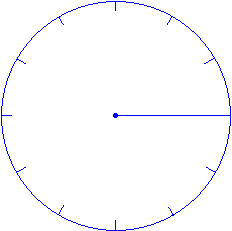Step 3:  Using a ruler and a pencil, draw lines joining each 30º mark to the centre of the circle to form 6 diameters.  The diagram thus obtained will have 12 parts as shown below.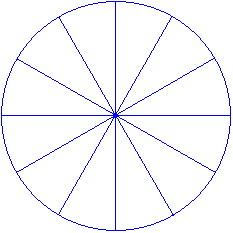Step 4:  Colour the parts as shown below.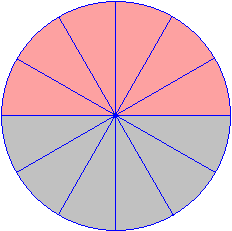Step 5:  Cut out the circle and then cut along the diameters so that all parts (i.e. sectors) are separated.

Step 6:  Arrange all of the sectors to make a shape that approximates a parallelogram as shown below.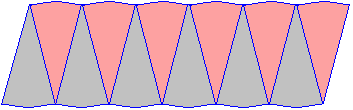Step 7:  Using a ruler, measure the base and the height of the approximate parallelogram obtained in Step 6.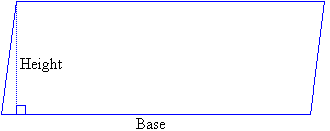###### Questions:

1.  Calculate the area of the figure in Step 6 by using the formula: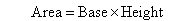2.  What is the area of the circle drawn in Step 1?

3.  It appears that there is a formula for calculating the area of a circle.  Can you discover it?

## Formula for the Area of a CircleFrom the above activity, it is clear that by arranging the sectors of the circle as a parallelogram that: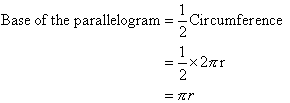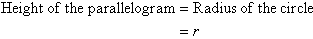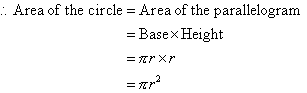###### Remember:

The area, A, of a circle is given by the following formula where r is the radius of the circle: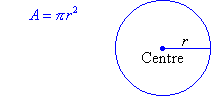#### Example 8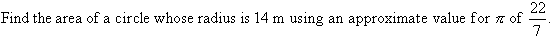##### Solution: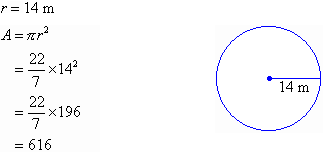So, the area is 616 m2.

###### Note:

To find the area of a region enclosed within a plane figure, draw a diagram and write an appropriate formula.  Then substitute the given values and use a calculator, if necessary, to obtain the required area.

#### Example 9

Find the area of a circle of whose diameter is 11 cm using π = 3.14.  Round your answer to 2 decimal places.

##### Solution: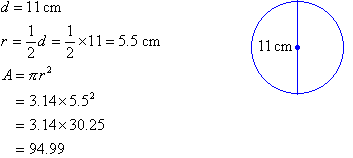So, the area is 94.99 cm2.

Copyright © 2000-2019 mathsteacher.com Pty Ltd.  All rights reserved.
Australian Business Number 53 056 217 611

Copyright instructions for educational institutions

Please read the Terms and Conditions of Use of this Website and our Privacy and Other Policies.
If you experience difficulties when using this Website, tell us through the feedback form or by phoning the contact telephone number.